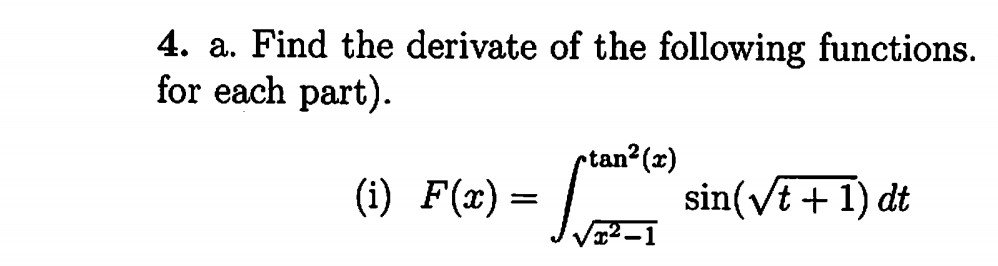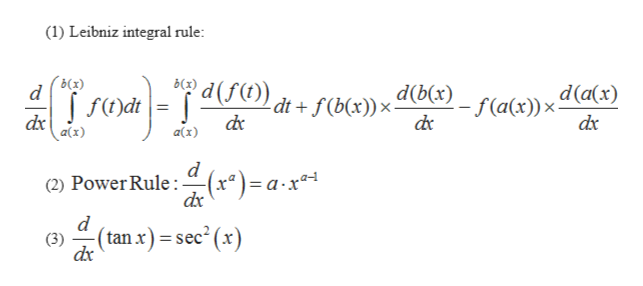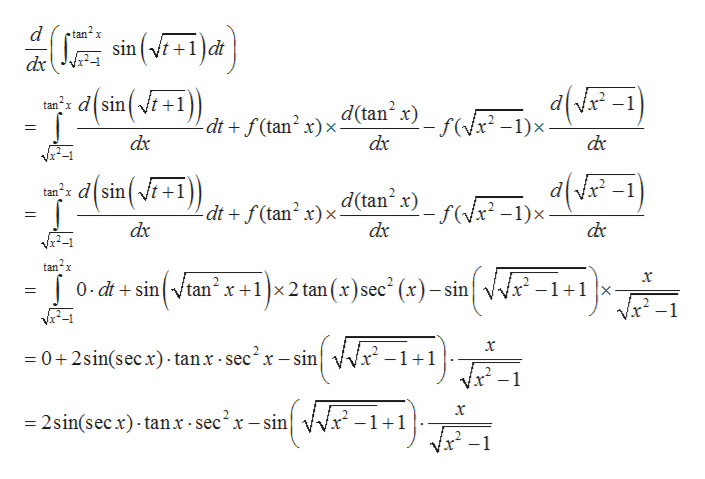# 4. a. Find the derivate of the following functionsfor each part)ptan2(x)sin(vt1) dtFte)-

Question
4 viewshelp_outlineImage Transcriptionclose4. a. Find the derivate of the following functions for each part) ptan2(x) sin(vt1) dt Fte)- fullscreen
check_circle

Step 1

To find the derivative of given function.

Step 2

In order to find the derivative of given function, we use the following rules:help_outlineImage Transcriptionclose(1) Leibniz integral rule: Вс3) d d(a(x) 2- f(a(x))x- dc dt + f(b(x))x dx a(x) dx a(x) d (2) Power Rule (x*)= a-x* d (tan x) = sec2 (x) (3) dx fullscreen
Step 3

On solving, w...help_outlineImage Transcriptionclosed tan x sin t1dt dx tandsint+1)) d(tan x) f-1)x dtf(tan2 x)x dx dx dc trdsint+1 d(tan x)f-1)x- dt f(tan2 x)x dx dx tan2x (dë-1= x 0 dtsin tan x+1x2 tan (x)sec- (x)- sin -1+1 |x Vx2-1 x 0+2sin(sec). tan.x-sec2x- sin -1+1 _ x -2sin(secx) tanx sec2x-sin 1 1 -1 fullscreen

### Want to see the full answer?

See Solution

#### Want to see this answer and more?

Solutions are written by subject experts who are available 24/7. Questions are typically answered within 1 hour.*

See Solution
*Response times may vary by subject and question.
Tagged in

### Calculus